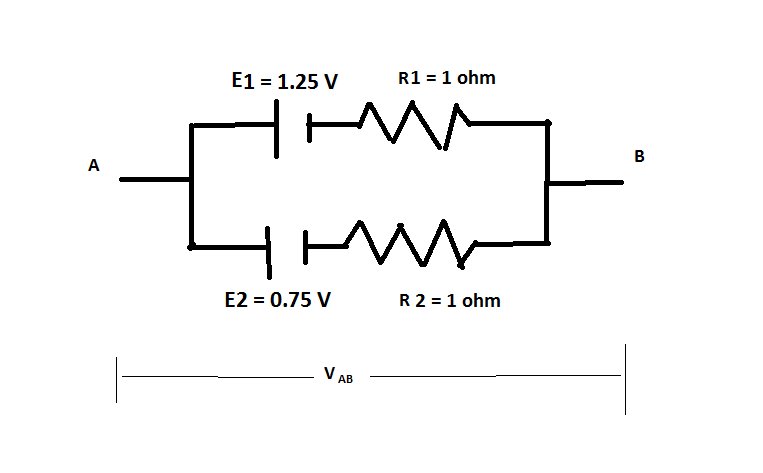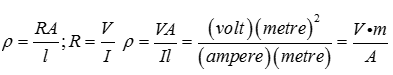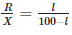Courses

# Test: Current Electricity- 1

## 30 Questions MCQ Test Physics Class 12 | Test: Current Electricity- 1

Description
This mock test of Test: Current Electricity- 1 for JEE helps you for every JEE entrance exam. This contains 30 Multiple Choice Questions for JEE Test: Current Electricity- 1 (mcq) to study with solutions a complete question bank. The solved questions answers in this Test: Current Electricity- 1 quiz give you a good mix of easy questions and tough questions. JEE students definitely take this Test: Current Electricity- 1 exercise for a better result in the exam. You can find other Test: Current Electricity- 1 extra questions, long questions & short questions for JEE on EduRev as well by searching above.
QUESTION: 1

### According to Kirchhoff’s Loop Rule

Solution:

Kirchhoff’s loop rule is based on the principle of conservation of energy. The work done in transporting a charge in a closed loop is zero. The algebraic sum ( since potential differences can be both positive and negative) of potential differences around any closed loop is always zero.

QUESTION: 2

### The Wheatstone bridge is balanced for four resistors R1,R2,R3 and R4 with a cell of emf 1.46 V. The cell is now replaced by another cell of emf 1.08 V. To obtain the balance again

Solution:

The balance point of the Wheatstone’s bridge is determined by the ratio of the resistances. The change in the emf of the external battery will have no effect on the balance point.

QUESTION: 3

### Resistance of a conductor is

Solution:

According to Ohm’s law, V = IR. Therefore R= V/I

QUESTION: 4

Two cells of emf 1.25V , 0.75V and each of internal resistance 1Ω are connected in parallel. The effective emf will be

Solution:We want the Voltage difference VAB.
let A and B be open and not connected to any thing.
There is a current that flows from cell of larger emf to the cell of small emf.
Call that current as  I Amperes.

1.25 V - 0.75 V - I * R2 - I * R1 = 0

I = 0.5 / (R1+R2)
VAB =  -0.75 - I * R2 =  - 0.75 - 0.5 * R2 / (R1+R2)

= - (0.75 R1 + 1.25 R2) / (R1+R2)

= - ().75 * 1 + 1.25 * 1) / (1 + 1)  volts

=  - 1 volts

QUESTION: 5

Flow of charges in direction of electrons is called

Solution:

The flow of electrons is termed electronic current. Electrons flow from the negative terminal to the positive.

QUESTION: 6

In the measurement of resistance by a metre bridge, the current is necessarily reversed through the bridge wire to eliminate

Solution:

The connecting metal strips include a small resistance in the circuit called the end resistance.On reversing the current, the end resistance tends to get cancelled out.

QUESTION: 7

If the length of the filament of a heater is reduced by 10% the power of the heater will

Solution:

Power P= V2/R​
If length reduced 10% then new resistance of filament will be R′.
R′=R−10% of R
R′=0.9R
Now new power of heater is P2​
P2​= V2​​/R′ = V2/0.9R​​=1.1P
% increase power=11%

QUESTION: 8

Resistance of a conductor is

Solution:

According to Ohm’s law, V=I R. Therefore R=V/I

QUESTION: 9

According to Ohm's law

Solution:

Ohm’s law states I is proportional to V.This holds good at steady temperatures and for the flow of constant current.

QUESTION: 10

Potentiometer is

Solution:

Potentiometer is a long wire of uniform cross section made of manganin.

QUESTION: 11

The unit of current is

Solution:

1. Newton is SI Unit of Force
2. Joule is SI Unit of Work Done
3. Coulomb is SI Unit of Charge
Hence, the unit of current is Ampere.

QUESTION: 12

A Wheatstone bridge ABCD is balanced with a galvanometer between the points B and D. At balance the resistance between the points B and D is :

Solution:

No current passes through the Galvanometer in the bridge balance condition.Therefore, the resistance between B and D is infinite.

QUESTION: 13

The instrument for the accurate measurement of the e.m.f of a cell is

Solution:
• Both potentiometer and voltmeter are devices to measure potential difference.
• EMF is the terminal p.d between the electrodes of a cell in open circuit, i.e., when no current is drawn from it.
• Potentiometer measures the potential difference using null deflection method, where no current is drawn from the cell; whereas voltmeter needs a small current to show deflection.
• So, accurate measurement of p.d is done using a potentiometer.
QUESTION: 14

Electromotive force is

Solution:
• An electric field exists in the electrolyte between the positive and negative terminals of the battery.
• In the external circuit, the current flows from the positive electrode to the negative electrode.
• To maintain continuity, in the electrolyte, the current (positive charges) flow from the negative electrode (lower potential) to the positive electrode (higher potential).
• Work done by the source in taking unit positive charge from lower to higher potential is called electromotive force.
QUESTION: 15

Drift is the random motion of the charged particles within a conductor,

Solution:

The electrons in a conductor have random velocities and when an electric field is applied, they suffer repeated collisions and in the process move with a small average velocity, opposite to the direction of the field. This is equivalent to positive charge flowing in the direction of the field.

QUESTION: 16

Unit of Resistivity is

Solution:QUESTION: 17

Potentiometer measures the potential difference more accurately than a voltmeter, because

Solution:

Potentiometer measures the potential difference using null deflection method, where no current is drawn from the cell; whereas voltmeter needs a small current to show deflection. So, accurate measurement of p.d is done using a potentiometer.

QUESTION: 18

Meter Bridge is based on the principle of

Solution:

Meter bridge is the application of wheatstone’s bridge. A Wheatstone bridge is an electrical network which is used to calculate the value of unknown resistance.

QUESTION: 19

A piece of copper and another of germanium are cooled from room temperature to 80K. The resistance

Solution:
• Copper is a conductor and we know that for conductors, resistance is directly proprtional to temperature. Therefore on decreasing temperature resistance also decreases.
• Whereas, germanium is a semiconductor and for semiconductors, resistance is inversely proportional to temperature.
• So on decreasing temperature resistance increases.
QUESTION: 20

Unit of power is

Solution:

Power is rate of doing work.P=W/t.The unit of power is J/s which is also called watt.

QUESTION: 21

In a potentiometer experiment, for measuring internal resistance of a cell, the balance point has been obtained on the fourth wire. The balance point can be shifted to fifth wire by

Solution:

If the current due to the auxillary battery is decreased, the potential gradient will be decreases, so the balancing length increases.Thus null point will move to fifth wire.

QUESTION: 22

Meter Bridge is used to

Solution:

With a known resistance in one of the gaps, the meter bridge is used to determine the value of unknown resistance by the formula.QUESTION: 23

According to Kirchhoff’s Junction Rule

Solution:

Kirchhoff’s junction rule is based on the principle of conservation of charge. For steady currents, there can be no accumulation of charge in a junction. The currents entering a junction bring charge to the junction and the currents leaving a junction carry away the charge brought to the junction.

QUESTION: 24

Ammeter is always used

Solution:

Ammeter is a device used to measure current. Since it has to allow the complete current flowing in the circuit through it, it has to be connected in series. For this reason, ammeters have very low values of resistances so that they do not add to the value of resistance connected in the circuit.

QUESTION: 25

The sensitivity of the potentiometer can be increased by:

Solution:
• A potentiometer is considered to be sensitive if the potential gradient dV/dl is low.
• Such a potentiometer can measure very small changes in potential difference.
• Increasing the length of the potentiometer wire decreases the potential gradient. Its sensitivity increases. Increasing potential gradient decreases the sensitivity.
• Increasing the emf of the primary cell and by decreasing the length, potential gradient increases.
QUESTION: 26

Direction of the conventional current

Solution:

Current flows in a conductor due to the flow of negatively charged electrons. However, the direction of conventional current is taken to be opposite to the direction of flow of electrons. It can therefore be considered as the direction in which positive charges move.

QUESTION: 27

Kirchhoff’s second law is a consequence of

Solution:

Kirchhoff's second law, also known as the Kirchhoff's voltage law (KVL) states that the sum of all voltages around a closed loop in any circuit must be equal to zero. This again is a consequence of conservation of energy.

QUESTION: 28

A battery is connected with a potentiometer wire. The internal resistance of the battery is negligible. If the length of the potentiometer wire of the same material and radius is doubled then

Solution:

Potential gradient Is given by V/l. If V and l are constant, the potential gradient also remains constant. The change in the radius will cause a change in the current. This does not change the potential gradient.

QUESTION: 29

In a meter bridge experiment a balance point is obtained at a distance of 60 cm from the left end when unknown resistance R is in a left gap and 8 ohms resistor is connected in the right gap. When the position of R and 8 ohm resistor is interchanged the balance point will be at distance of

Solution:

60/40 = l/100-l, = R/8

so R=12

now changed position 8 is proportional to l and 12 to (100-l)

8/12 = l/100-l

l = 40ohm

QUESTION: 30

A Wheatstone bridge is balanced for four resistors R1, R2, R3 and R4 with a Lech lanche cell between A and C and a galvanometer between B and D. The positions of the cell and the galvanometer are interchanged. The balance will

Solution:

The balance point will not change . For balancing of wheatstone bridge four arms of resistors are responsible. Interchange the position of galvanometer and cell does not affect balancing of wheatstone bridge.